# Reverse Cuthill Mckee Algorithm

The Cuthill-Mckee algorithm is used for reordering of a symmetric square matrix. It is based on Breadth First Search algorithm of a graph, whose adjacency matrix is the sparsified version of the input square matrix.

The ordering is frequently used when a matrix is to be generated whose rows and columns are numbered according to the numbering of the nodes. By an appropriate renumbering of the nodes, it is often possible to produce a matrix with a much smaller bandwidth.
Sparsified version of a matrix is a matrix in which most of the elements are zero.

The Reverse Cuthill-Mckee Algorithm is the same as the Cuthill-Mckee algorithm, the only difference is that the final indices obtained using the Cuthill-Mckee algorithm are reversed in the Reverse Cuthill-Mckee Algorithm.

Below are the steps of Reverse Cuthill-Mckee algorithm:

1. Instantiate an empty queue Q and empty array for permutation order of the objects R.
2. S1: We first find the object with minimum degree whose index has not yet been added to R. Say, object corresponding to pth row has been identified as the object with a minimum degree. Add p to R.
3. S2: As an index is added to R, and add all neighbors of the corresponding object at the index,
in increasing order of degree, to Q. The neighbors are nodes with non-zero value amongst the
non-diagonal elements in the pth row.
4. S3: Extract the first node in Q, say C. If C has not been inserted in R, add it to R, add to Q the neighbors of C in increasing order of degree.
5. S4: If Q is not empty, repeat S3.
6. S5: If Q is empty, but there are objects in the matrix which have not been included in R, start from S1, once again. (This could happen if there are disjoint graphs)
7. S6: Terminate this algorithm once all objects are included in R.
8. S7: Finally, reverse the indices in R, i.e. (swap(R[i], R[P-i+1])).

Degree : Definition of a degree is not constant, it changes according to the dataset you are working with. For the example given below, the degree of a node is defined as the sum of non-diagonal elements in the corresponding row.

Generalized definition of the degree of a node ‘A’ is the number of nodes connected to ‘A’.

Example:

```Given a symetric matrix:
| 0.0    0.78    0.79    0.8     0.23  |
| 0.9    0.0     0.43    0.771   0.752 |
| 0.82   0.0     0.0     0.79    0.34  |
| 0.8    0.8     0.8     0.0     0.8   |
| 0.54   0.97    0.12    0.78    0.0   |

Degree here is defined as sum of non-diagonal
elements in the corresponding row.
Sparsification for a matrix is defined as,
if the element of the matrix at i, j has a value
less than 0.75 its made to 0 otherwise its made to 1.
```

Matrix after Sparsification: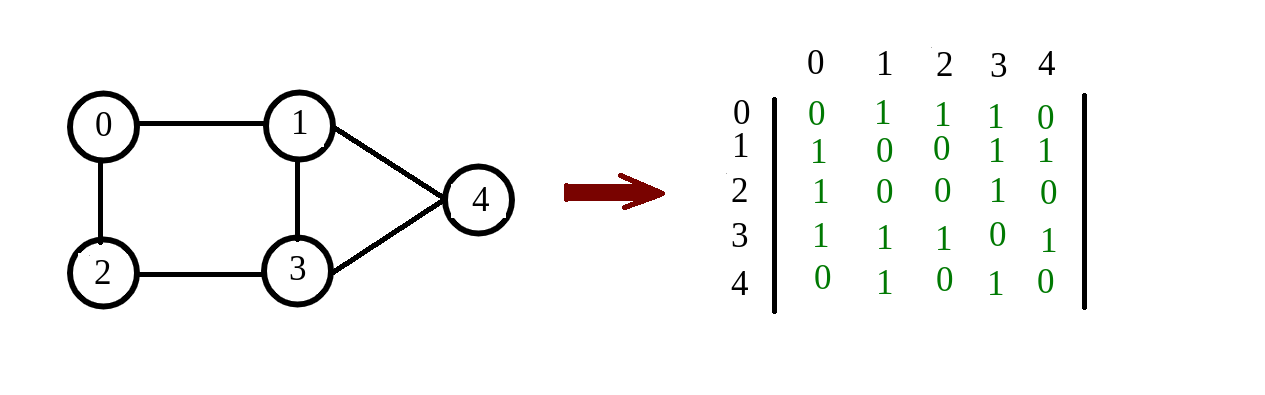```Degree of node 0 = 2.6
Degree of node 1 = 2.803
Degree of node 2 = 2.55
Degree of node 3 = 3.2
Degree of node 4 = 2.41

Permutation order of objects (R) : 0 2 1 3 4
```

The new permutation order is just ordering of the nodes, i.e. convert node ‘R[i]’ to node ‘i’ .
Therefore, convert node ‘R = 0’, to 0; node ‘R = 2’, to 1; node ‘R = 1’, to 2; node ‘R = 3’, to 3; and node ‘R = 4’, to 4;

Let’s take a bigger example to understand the result of the reordering:

```Give a adjacency matrix :

| 0   1   0   0   0   0   1   0   1   0 |
| 1   0   0   0   1   0   1   0   0   1 |
| 0   0   0   0   1   0   1   0   0   0 |
| 0   0   0   0   1   1   0   0   1   0 |
| 0   1   1   1   0   1   0   0   0   1 |
| 0   0   0   1   1   0   0   0   0   0 |
| 1   1   1   0   0   0   0   0   0   0 |
| 0   0   0   0   0   0   0   0   1   1 |
| 1   0   0   1   0   0   0   1   0   0 |
| 0   1   0   0   1   0   0   1   0   0 |

Degree of node 'A' is defined as number of
nodes connected to 'A'
```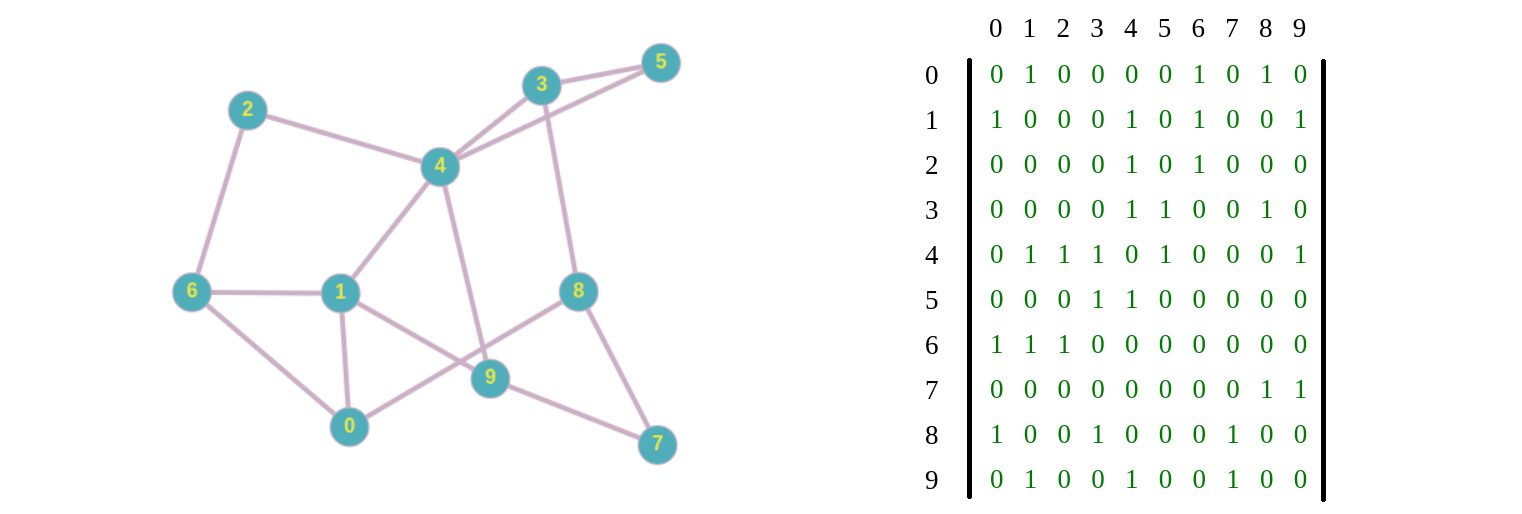```Output :
Permutation order of objects (R) : 7 8 3 5 9 0 1 4 6 2
```

Now convert node ‘R[i]’ to node ‘i’
So the graph becomes: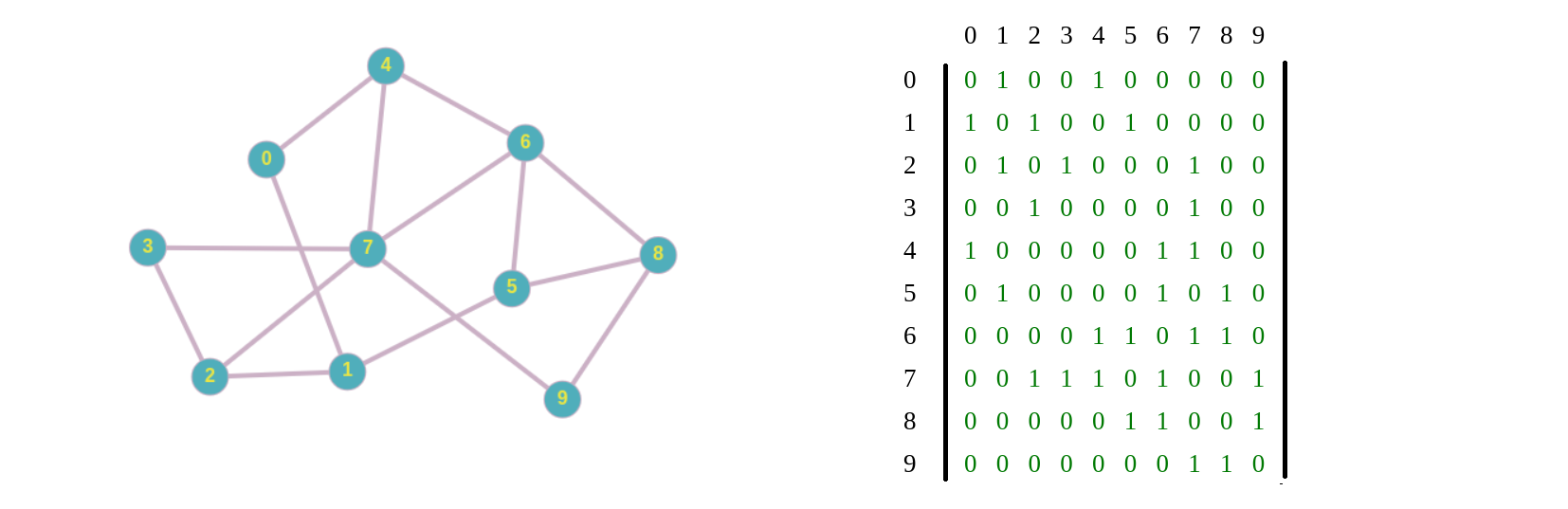The result of reoerdering can be seen by the adjacency matrix of the two graph: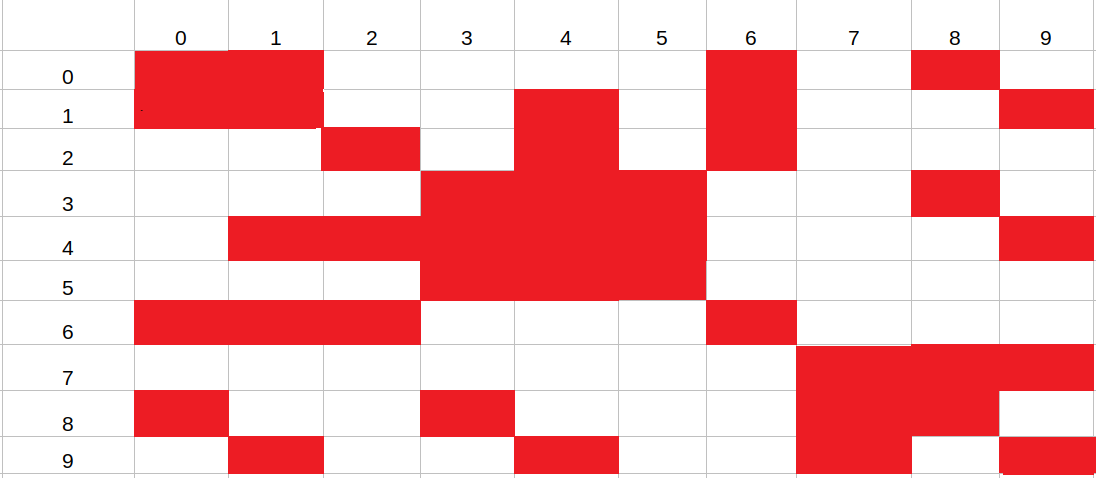Original Matrix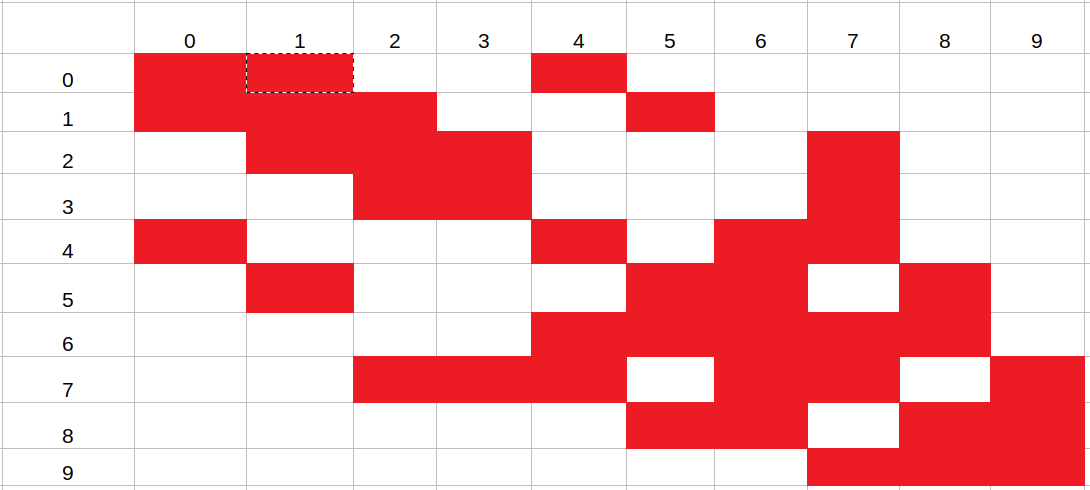RCM Reordered Matrix

From here we can clearly see that how the Cuthill-Mckee algorithm helps in the reordering of a square matrix into a non distributed matrix.

Below is the implementation of the above algorithm. Taking the general definition of degree.

 `// C++ program for Implementation of ` `// Reverse Cuthill Mckee Algorithm ` ` `  `#include ` `using` `namespace` `std; ` ` `  `vector<``double``> globalDegree; ` ` `  `int` `findIndex(vector > a, ``int` `x) ` `{ ` `    ``for` `(``int` `i = 0; i < a.size(); i++) ` `        ``if` `(a[i].first == x) ` `            ``return` `i; ` `    ``return` `-1; ` `} ` ` `  `bool` `compareDegree(``int` `i, ``int` `j) ` `{ ` `    ``return` `::globalDegree[i] - ::globalDegree[j]; ` `} ` ` `  `template` `<``typename` `T> ` `ostream& operator<<(ostream& out, vector ``const``& v) ` `{ ` `    ``for` `(``int` `i = 0; i < v.size(); i++) ` `        ``out << v[i] << ``' '``; ` `    ``return` `out; ` `} ` ` `  `class` `ReorderingSSM { ` `private``: ` `    ``vector > _matrix; ` ` `  `public``: ` `    ``// Constructor and Destructor ` `    ``ReorderingSSM(vector > m) ` `    ``{ ` `        ``_matrix = m; ` `    ``} ` ` `  `    ``ReorderingSSM() {} ` `    ``~ReorderingSSM() {} ` ` `  `    ``// class methods ` ` `  `    ``// Function to generate degree of all the nodes ` `    ``vector<``double``> degreeGenerator() ` `    ``{ ` ` `  `        ``vector<``double``> degrees; ` ` `  `        ``for` `(``int` `i = 0; i < _matrix.size(); i++) { ` `            ``double` `count = 0; ` `            ``for` `(``int` `j = 0; j < _matrix.size(); j++) { ` `                ``count += _matrix[i][j]; ` `            ``} ` ` `  `            ``degrees.push_back(count); ` `        ``} ` ` `  `        ``return` `degrees; ` `    ``} ` ` `  `    ``// Implementation of Cuthill-Mckee algorithm ` `    ``vector<``int``> CuthillMckee() ` `    ``{ ` `        ``vector<``double``> degrees = degreeGenerator(); ` ` `  `        ``::globalDegree = degrees; ` ` `  `        ``queue<``int``> Q; ` `        ``vector<``int``> R; ` `        ``vector > notVisited; ` ` `  `        ``for` `(``int` `i = 0; i < degrees.size(); i++) ` `            ``notVisited.push_back(make_pair(i, degrees[i])); ` ` `  `        ``// Vector notVisited helps in running BFS ` `        ``// even when there are dijoind graphs ` `        ``while` `(notVisited.size()) { ` ` `  `            ``int` `minNodeIndex = 0; ` ` `  `            ``for` `(``int` `i = 0; i < notVisited.size(); i++) ` `                ``if` `(notVisited[i].second < notVisited[minNodeIndex].second) ` `                    ``minNodeIndex = i; ` ` `  `            ``Q.push(notVisited[minNodeIndex].first); ` ` `  `            ``notVisited.erase(notVisited.begin()  ` `                                    ``+ findIndex(notVisited, ` `                                                ``notVisited[Q.front()].first)); ` ` `  `            ``// Simple BFS ` `            ``while` `(!Q.empty()) { ` ` `  `                ``vector<``int``> toSort; ` ` `  `                ``for` `(``int` `i = 0; i < _matrix.size(); i++) { ` `                    ``if` `(i != Q.front() && _matrix[Q.front()][i] == 1 ` `                        ``&& findIndex(notVisited, i) != -1) { ` `                        ``toSort.push_back(i); ` `                        ``notVisited.erase(notVisited.begin()  ` `                                           ``+ findIndex(notVisited, i)); ` `                    ``} ` `                ``} ` ` `  `                ``sort(toSort.begin(), toSort.end(), compareDegree); ` ` `  `                ``for` `(``int` `i = 0; i < toSort.size(); i++) ` `                    ``Q.push(toSort[i]); ` ` `  `                ``R.push_back(Q.front()); ` `                ``Q.pop(); ` `            ``} ` `        ``} ` ` `  `        ``return` `R; ` `    ``} ` ` `  `    ``// Implementation of reverse Cuthill-Mckee algorithm ` `    ``vector<``int``> ReverseCuthillMckee() ` `    ``{ ` ` `  `        ``vector<``int``> cuthill = CuthillMckee(); ` ` `  `        ``int` `n = cuthill.size(); ` ` `  `        ``if` `(n % 2 == 0) ` `            ``n -= 1; ` ` `  `        ``n = n / 2; ` ` `  `        ``for` `(``int` `i = 0; i <= n; i++) { ` `            ``int` `j = cuthill[cuthill.size() - 1 - i]; ` `            ``cuthill[cuthill.size() - 1 - i] = cuthill[i]; ` `            ``cuthill[i] = j; ` `        ``} ` ` `  `        ``return` `cuthill; ` `    ``} ` `}; ` ` `  `// Driver Code ` `int` `main() ` `{ ` `    ``int` `num_rows = 10; ` ` `  `    ``vector > matrix; ` ` `  `    ``for` `(``int` `i = 0; i < num_rows; i++) { ` `        ``vector<``double``> datai; ` ` `  `        ``for` `(``int` `j = 0; j < num_rows; j++) ` `            ``datai.push_back(0.0); ` ` `  `        ``matrix.push_back(datai); ` `    ``} ` ` `  `    ``// This is the test graph, ` `    ``// check out the above graph photo ` `    ``matrix = { 0, 1, 0, 0, 0, 0, 1, 0, 1, 0 }; ` `    ``matrix = { 1, 0, 0, 0, 1, 0, 1, 0, 0, 1 }; ` `    ``matrix = { 0, 0, 0, 0, 1, 0, 1, 0, 0, 0 }; ` `    ``matrix = { 0, 0, 0, 0, 1, 1, 0, 0, 1, 0 }; ` `    ``matrix = { 0, 1, 1, 1, 0, 1, 0, 0, 0, 1 }; ` `    ``matrix = { 0, 0, 0, 1, 1, 0, 0, 0, 0, 0 }; ` `    ``matrix = { 1, 1, 1, 0, 0, 0, 0, 0, 0, 0 }; ` `    ``matrix = { 0, 0, 0, 0, 0, 0, 0, 0, 1, 1 }; ` `    ``matrix = { 1, 0, 0, 1, 0, 0, 0, 1, 0, 0 }; ` `    ``matrix = { 0, 1, 0, 0, 1, 0, 0, 1, 0, 0 }; ` ` `  `    ``ReorderingSSM m(matrix); ` ` `  `    ``vector<``int``> r = m.ReverseCuthillMckee(); ` ` `  `    ``cout << ``"Permutation order of objects: "` `<< r << endl; ` ` `  `    ``return` `0; ` `} `

Output:

```Permutation order of objects: 7 8 3 5 9 0 1 4 6 2
```

My Personal Notes arrow_drop_upCheck out this Author's contributed articles.

If you like GeeksforGeeks and would like to contribute, you can also write an article using contribute.geeksforgeeks.org or mail your article to contribute@geeksforgeeks.org. See your article appearing on the GeeksforGeeks main page and help other Geeks.

Please Improve this article if you find anything incorrect by clicking on the "Improve Article" button below.

Article Tags :
Practice Tags :

Be the First to upvote.

Please write to us at contribute@geeksforgeeks.org to report any issue with the above content.